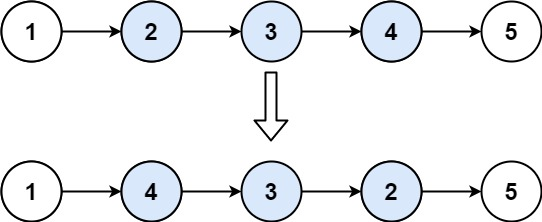# 92. Reverse Linked List II

Medium
Given the `head` of a singly linked list and two integers `left` and `right` where `left <= right`, reverse the nodes of the list from position `left` to position `right`, and return the reversed list.
Example 1:Input: head = [1,2,3,4,5], left = 2, right = 4
Output:
[1,4,3,2,5]
Example 2:
Input: head = , left = 1, right = 1
Output:

Constraints:
• The number of nodes in the list is `n`.
• `1 <= n <= 500`
• `-500 <= Node.val <= 500`
• `1 <= left <= right <= n`
Follow up: Could you do it in one pass?

### 解題

Runtime: 0 ms, faster than 100%
Memory Usage: 2.1 MB, less than 16.81%
/**
* type ListNode struct {
* Val int
* Next *ListNode
* }
*/
func reverseBetween(head *ListNode, left int, right int) *ListNode {
res := &ListNode{}
r := res
stack := make([]int, 0)
count := 1
if count == left {
break
}
res = res.Next
count++
}
// 要反轉的部分放到 stack
if count == right {
break
}
count++